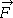# What is the magnitude of the acceleration of cylinder's com?

## Homework Statement

In the figure below, a constant horizontal forceapp of magnitude 18 N is applied to a uniform solid cylinder by fishing line wrapped around the cylinder. The mass of the cylinder is 19 kg, its radius is 0.11 m, and the cylinder rolls smoothly on the horizontal surface.
(a) What is the magnitude of the acceleration of the center of mass of the cylinder?

## The Attempt at a Solution

I'm confused... I feel like I could use torque — I could set the bottom point as the origin, and then tau_net = 18 x 2 x 0.11 + F_cm x 0.11.
However, I couldn't really get further than that and I am really confused. Could someone help me?

#### Attachments

Taking torque about the bottom point is a convenient way of eliminating
the frictional force.
Are you familiar with the parallel axis theorem?
You can also apply Newton's equations about the center of mass
to eliminate the frictional force.

@J Hann — what's the parallel axis theorem?

@J Hann — what's the parallel axis theorem?
The moment of inertia I of a body about any axis is equal to the moment of inertia
I CM of the body about a parallel axis through its center of mass plus the mass M of the
body times the square of the perpendicular distance L between the axes:
I = Icm + M L^2.
For a cylinder about an edge I = 1/2 M R^2 + M R^2 = 3/2 M R^2

tau_net = 18 x 2 x 0.11 + F_cm x 0.11.

Taking the torque around the contact point was a very good idea. (Why?) However, your equation for the net torque is wrong. How many forces are there acting on the cylinder? And how many of those produce a torque about the contact point?